Publicité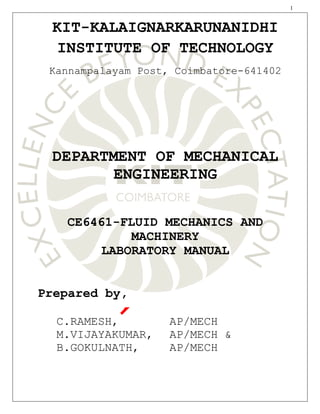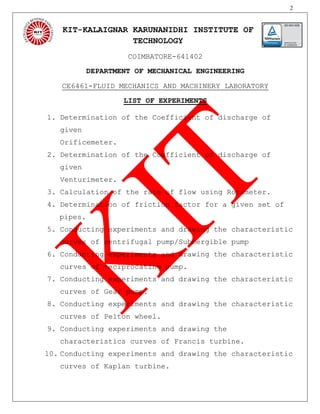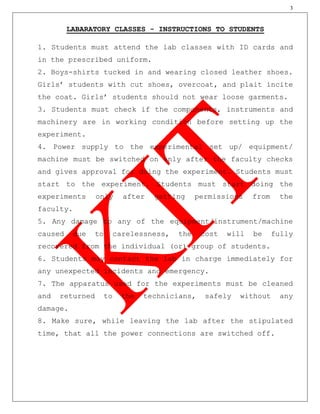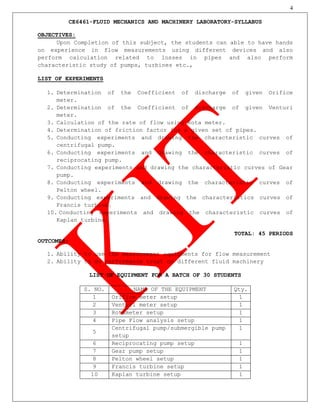Publicité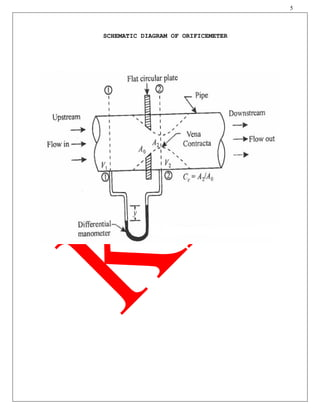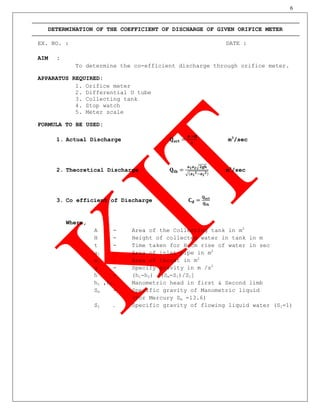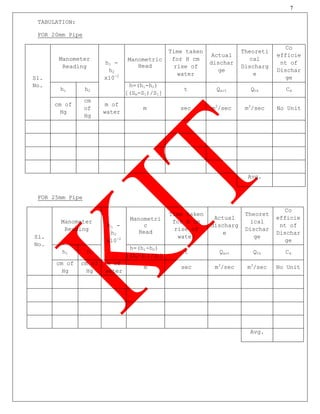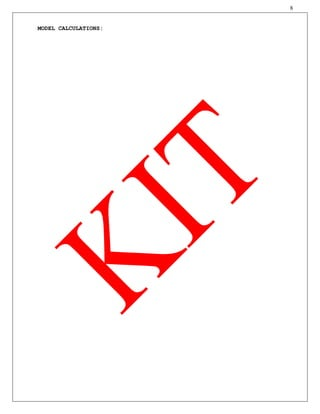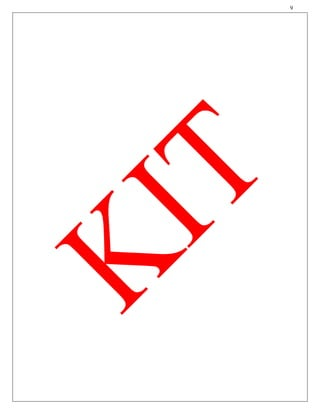Publicité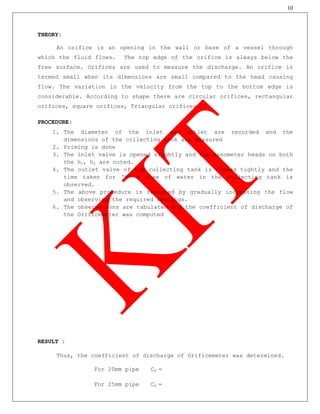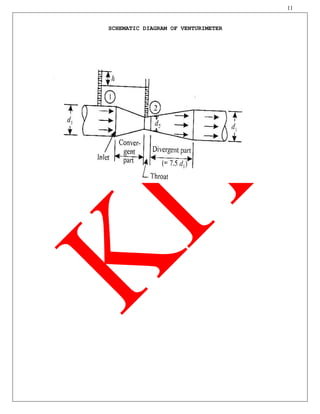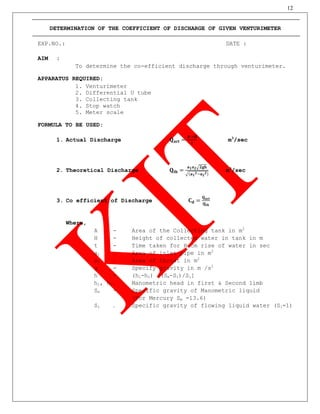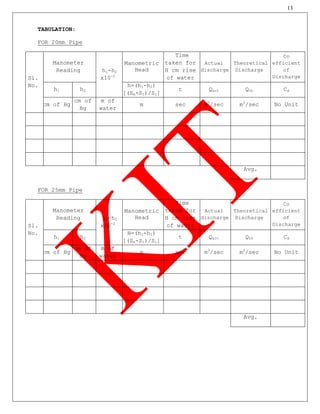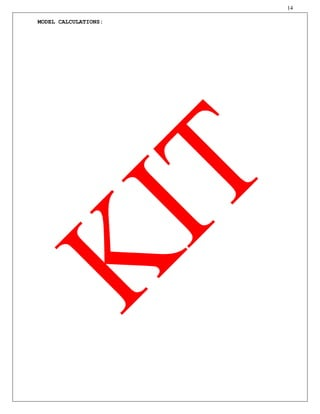Publicité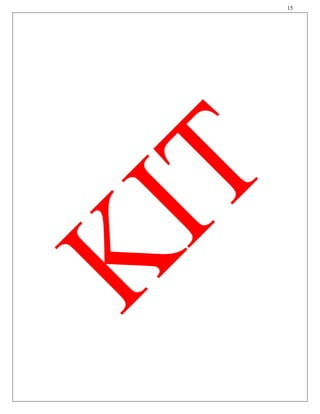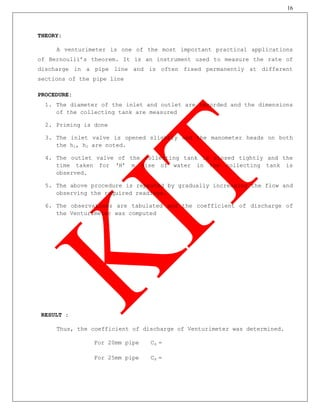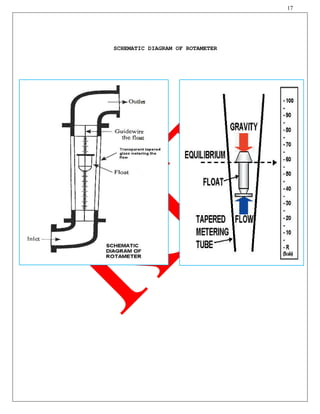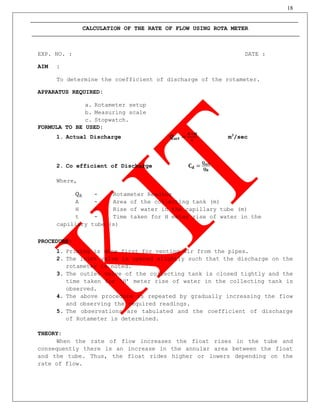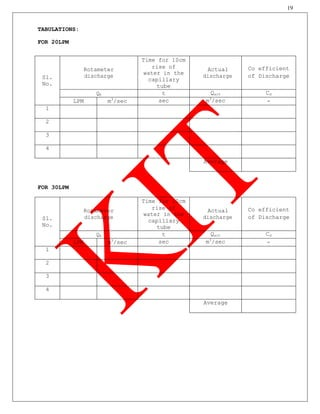Publicité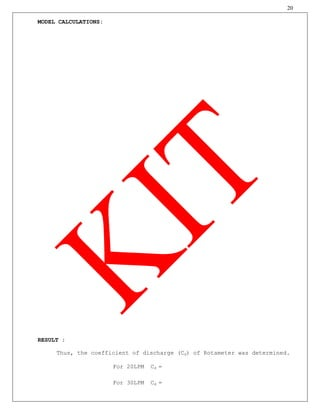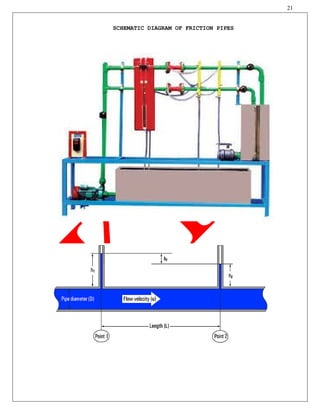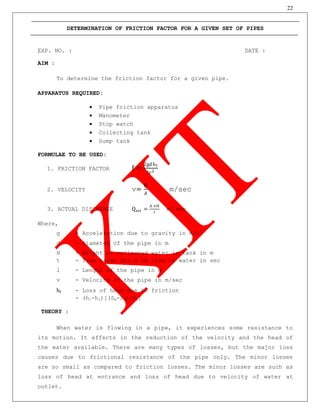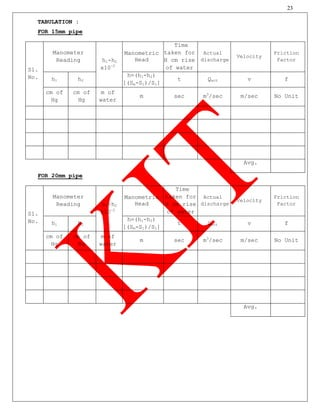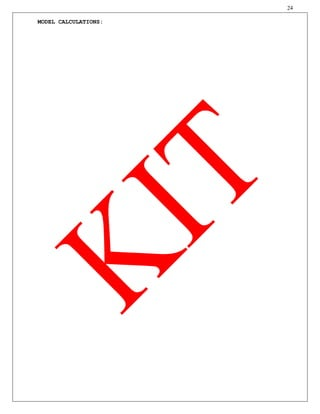Publicité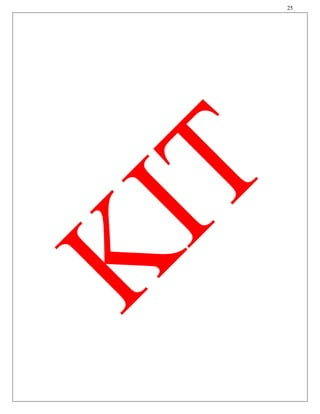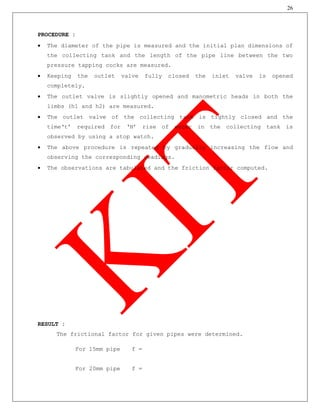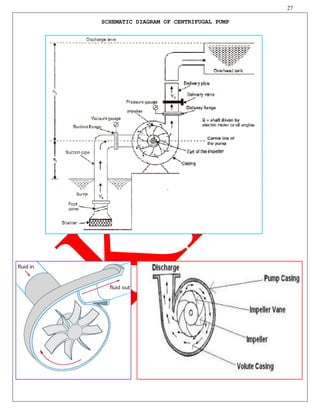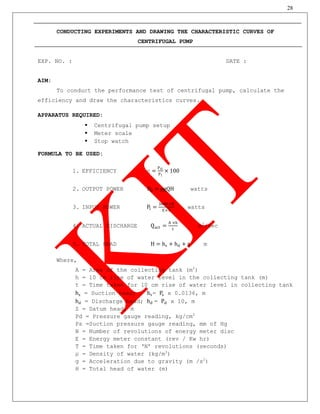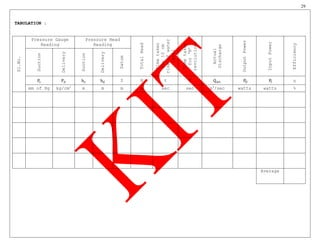Publicité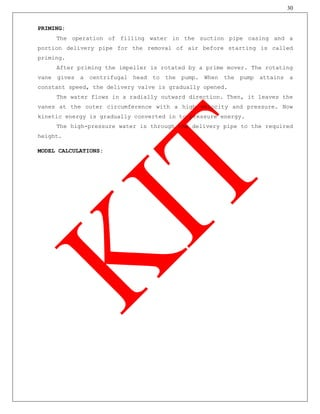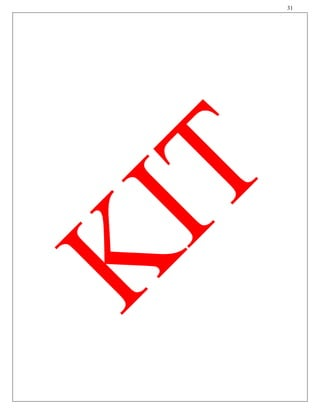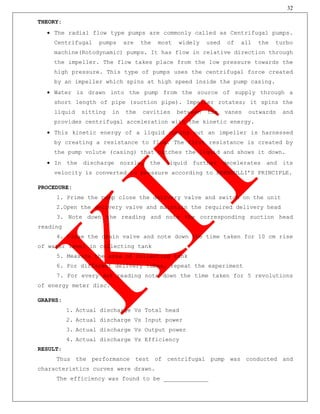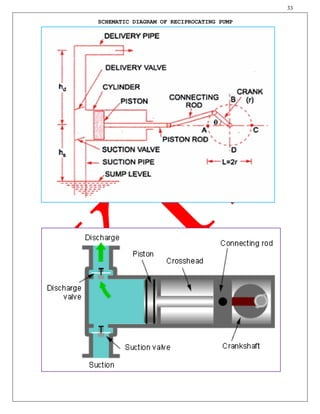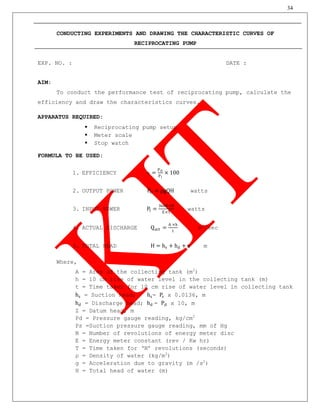Publicité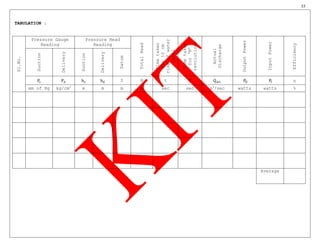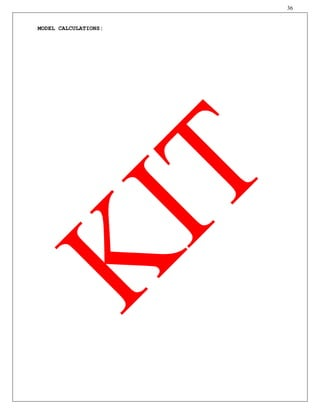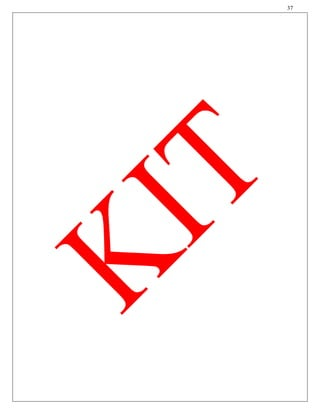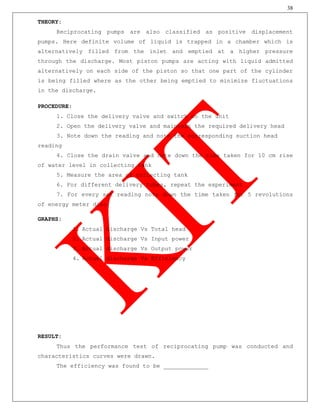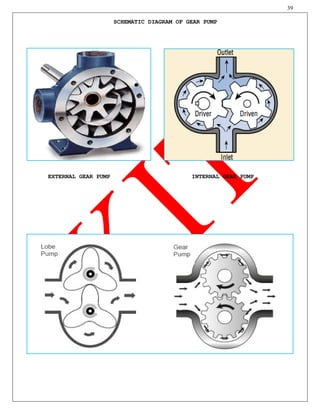Publicité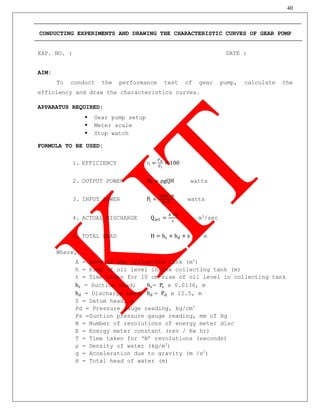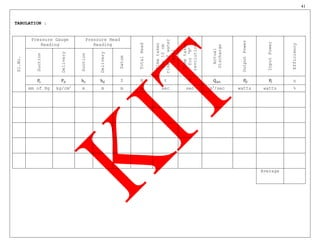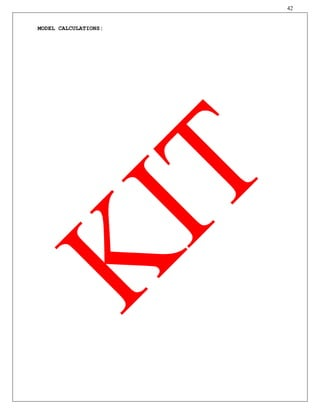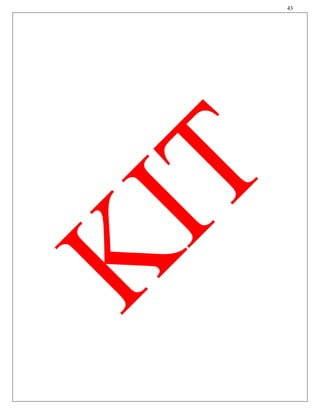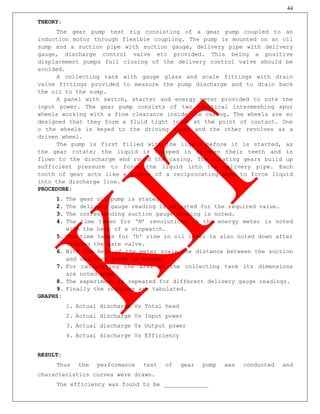Publicité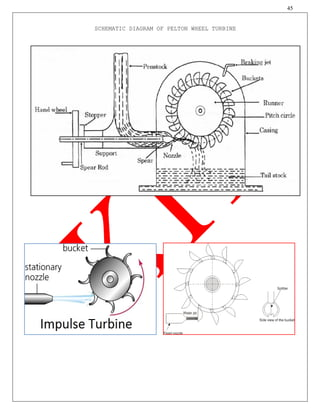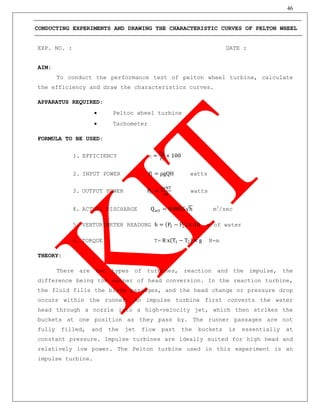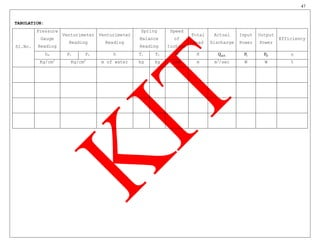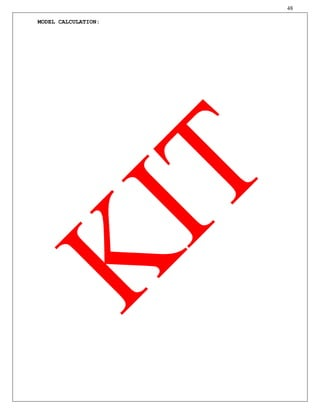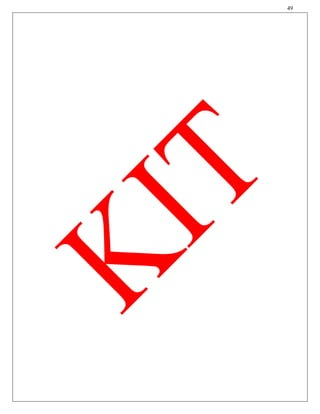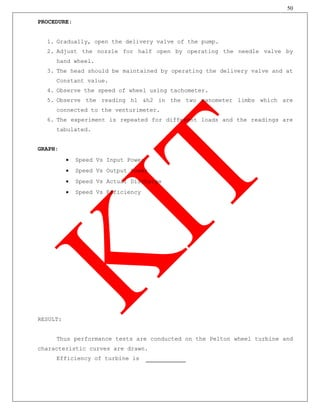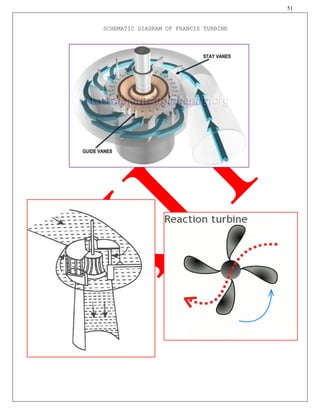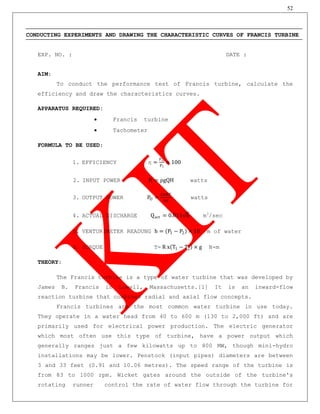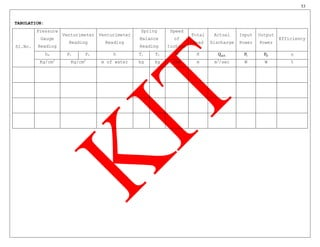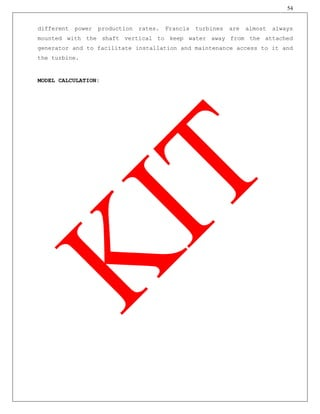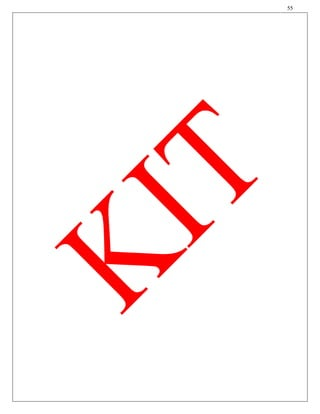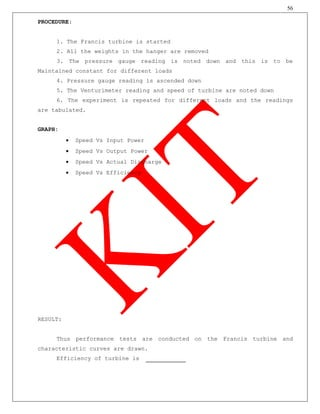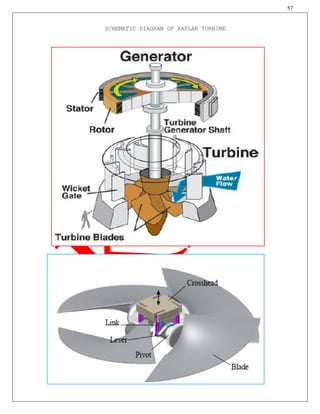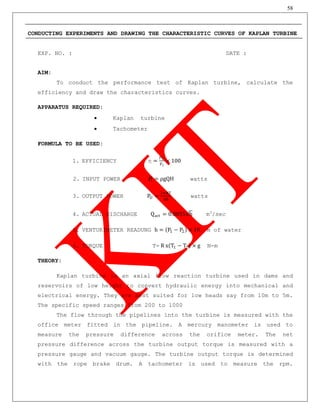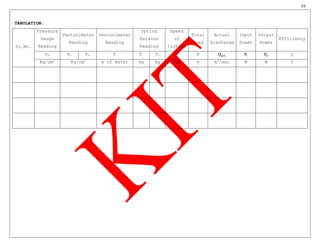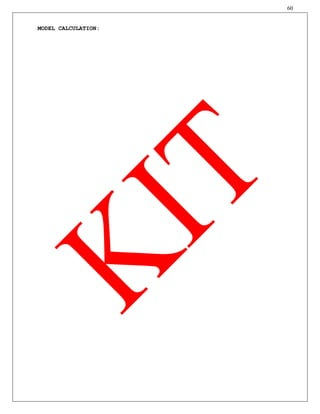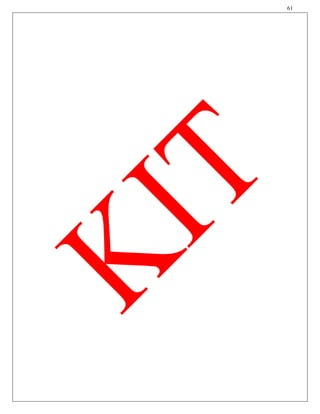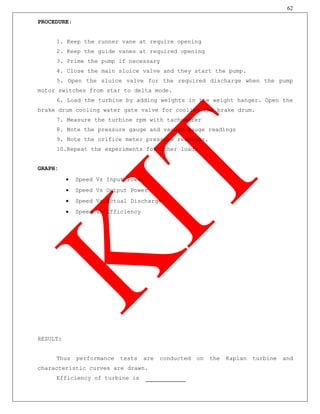Prochain SlideShare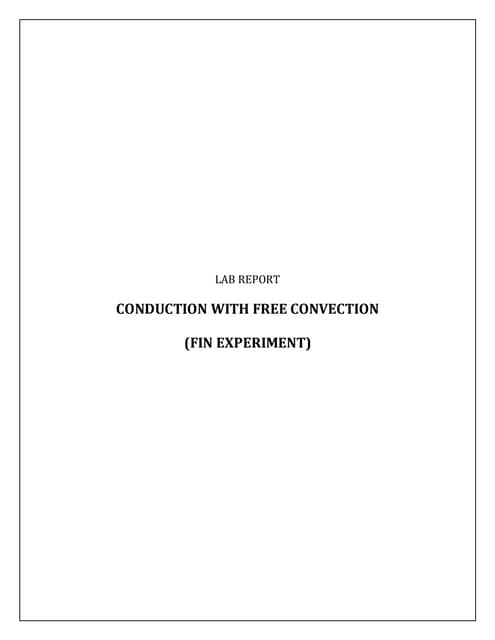Lab Report Conduction With Free Convection
Chargement dans ... 3
1 sur 62
Publicité

### Mech CE6461 FMM lab_manual

1. 1 KIT-KALAIGNARKARUNANIDHI INSTITUTE OF TECHNOLOGY Kannampalayam Post, Coimbatore-641402 DEPARTMENT OF MECHANICAL ENGINEERING CE6461-FLUID MECHANICS AND MACHINERY LABORATORY MANUAL Prepared by, C.RAMESH, AP/MECH M.VIJAYAKUMAR, AP/MECH & B.GOKULNATH, AP/MECH
2. 2 KIT-KALAIGNAR KARUNANIDHI INSTITUTE OF TECHNOLOGY COIMBATORE-641402 DEPARTMENT OF MECHANICAL ENGINEERING CE6461-FLUID MECHANICS AND MACHINERY LABORATORY LIST OF EXPERIMENTS 1. Determination of the Coefficient of discharge of given Orificemeter. 2. Determination of the Coefficient of discharge of given Venturimeter. 3. Calculation of the rate of flow using Rotameter. 4. Determination of friction factor for a given set of pipes. 5. Conducting experiments and drawing the characteristic curves of centrifugal pump/Submergible pump 6. Conducting experiments and drawing the characteristic curves of reciprocating pump. 7. Conducting experiments and drawing the characteristic curves of Gear pump. 8. Conducting experiments and drawing the characteristic curves of Pelton wheel. 9. Conducting experiments and drawing the characteristics curves of Francis turbine. 10. Conducting experiments and drawing the characteristic curves of Kaplan turbine.
3. 3 LABARATORY CLASSES - INSTRUCTIONS TO STUDENTS 1. Students must attend the lab classes with ID cards and in the prescribed uniform. 2. Boys-shirts tucked in and wearing closed leather shoes. Girls’ students with cut shoes, overcoat, and plait incite the coat. Girls’ students should not wear loose garments. 3. Students must check if the components, instruments and machinery are in working condition before setting up the experiment. 4. Power supply to the experimental set up/ equipment/ machine must be switched on only after the faculty checks and gives approval for doing the experiment. Students must start to the experiment. Students must start doing the experiments only after getting permissions from the faculty. 5. Any damage to any of the equipment/instrument/machine caused due to carelessness, the cost will be fully recovered from the individual (or) group of students. 6. Students may contact the lab in charge immediately for any unexpected incidents and emergency. 7. The apparatus used for the experiments must be cleaned and returned to the technicians, safely without any damage. 8. Make sure, while leaving the lab after the stipulated time, that all the power connections are switched off.
4. 4 CE6461-FLUID MECHANICS AND MACHINERY LABORATORY-SYLLABUS OBJECTIVES: Upon Completion of this subject, the students can able to have hands on experience in flow measurements using different devices and also perform calculation related to losses in pipes and also perform characteristic study of pumps, turbines etc., LIST OF EXPERIMENTS 1. Determination of the Coefficient of discharge of given Orifice meter. 2. Determination of the Coefficient of discharge of given Venturi meter. 3. Calculation of the rate of flow using Rota meter. 4. Determination of friction factor for a given set of pipes. 5. Conducting experiments and drawing the characteristic curves of centrifugal pump. 6. Conducting experiments and drawing the characteristic curves of reciprocating pump. 7. Conducting experiments and drawing the characteristic curves of Gear pump. 8. Conducting experiments and drawing the characteristic curves of Pelton wheel. 9. Conducting experiments and drawing the characteristics curves of Francis turbine. 10. Conducting experiments and drawing the characteristic curves of Kaplan turbine. TOTAL: 45 PERIODS OUTCOMES: 1. Ability to use the measurement equipments for flow measurement 2. Ability to do performance trust on different fluid machinery LIST OF EQUIPMENT FOR A BATCH OF 30 STUDENTS S. NO. NAME OF THE EQUIPMENT Qty. 1 Orifice meter setup 1 2 Venturi meter setup 1 3 Rotameter setup 1 4 Pipe Flow analysis setup 1 5 Centrifugal pump/submergible pump setup 1 6 Reciprocating pump setup 1 7 Gear pump setup 1 8 Pelton wheel setup 1 9 Francis turbine setup 1 10 Kaplan turbine setup 1
5. 5 SCHEMATIC DIAGRAM OF ORIFICEMETER
6. 6 DETERMINATION OF THE COEFFICIENT OF DISCHARGE OF GIVEN ORIFICE METER EX. NO. : DATE : AIM : To determine the co-efficient discharge through orifice meter. APPARATUS REQUIRED: 1. Orifice meter 2. Differential U tube 3. Collecting tank 4. Stop watch 5. Meter scale FORMULA TO BE USED: 1. Actual Discharge 𝐐 𝐚𝐜𝐭 = 𝐀 ×𝐇 𝐭 m3 /sec 2. Theoretical Discharge 𝐐𝐭𝐡 = 𝐚 𝟏 𝐚 𝟐 𝟐𝐠𝐡 𝐚 𝟏 𝟐−𝐚 𝟐 𝟐 m3 /sec 3. Co efficient of Discharge 𝐂 𝐝 = 𝐐 𝐚𝐜𝐭 𝐐 𝐭𝐡 Where, A - Area of the Collecting tank in m2 H - Height of collected water in tank in m t - Time taken for H cm rise of water in sec a1 - Area of inlet pipe in m2 a2 - Area of throat in m2 g - Specify gravity in m /s2 h - (h1-h2) [(Sm-S1)/S1] h1 ,h2 - Manometric head in first & Second limb Sm - Specific gravity of Manometric liquid (For Mercury Sm =13.6) S1 - Specific gravity of flowing liquid water (S1=1)
7. 7 TABULATION: FOR 20mm Pipe Sl. No. Manometer Reading h1 - h2 x10-2 Manometric Head Time taken for H cm rise of water Actual dischar ge Theoreti cal Discharg e Co efficie nt of Dischar ge h1 h2 h=(h1-h2) [(Sm-S1)/S1] t Qact Qth Cd cm of Hg cm of Hg m of water m sec m3 /sec m3 /sec No Unit Avg. FOR 25mm Pipe Sl. No. Manometer Reading h1 - h2 x10-2 Manometri c Head Time taken for H cm rise of water Actual discharg e Theoret ical Dischar ge Co efficie nt of Dischar ge h1 h2 h=(h1-h2) [(Sm-S1)/S1] t Qact Qth Cd cm of Hg cm of Hg m of water m sec m3 /sec m3 /sec No Unit Avg.
8. 8 MODEL CALCULATIONS:
9. 9
10. 10 THEORY: An orifice is an opening in the wall or base of a vessel through which the fluid flows. The top edge of the orifice is always below the free surface. Orifices are used to measure the discharge. An orifice is termed small when its dimensions are small compared to the head causing flow. The variation in the velocity from the top to the bottom edge is considerable. According to shape there are circular orifices, rectangular orifices, square orifices, Triangular orifices. PROCEDURE: 1. The diameter of the inlet and outlet are recorded and the dimensions of the collecting tank are measured 2. Priming is done 3. The inlet valve is opened slightly and the manometer heads on both the h1, h2 are noted. 4. The outlet valve of the collecting tank is closed tightly and the time taken for ‘H’ m rise of water in the collecting tank is observed. 5. The above procedure is repeated by gradually increasing the flow and observing the required readings. 6. The observations are tabulated and the coefficient of discharge of the Orificemeter was computed RESULT : Thus, the coefficient of discharge of Orificemeter was determined. For 20mm pipe Cd = For 25mm pipe Cd =
11. 11 SCHEMATIC DIAGRAM OF VENTURIMETER
12. 12 DETERMINATION OF THE COEFFICIENT OF DISCHARGE OF GIVEN VENTURIMETER EXP.NO.: DATE : AIM : To determine the co-efficient discharge through venturimeter. APPARATUS REQUIRED: 1. Venturimeter 2. Differential U tube 3. Collecting tank 4. Stop watch 5. Meter scale FORMULA TO BE USED: 1. Actual Discharge 𝐐 𝐚𝐜𝐭 = 𝐀 ×𝐇 𝐭 m3 /sec 2. Theoretical Discharge 𝐐𝐭𝐡 = 𝐚 𝟏 𝐚 𝟐 𝟐𝐠𝐡 𝐚 𝟏 𝟐−𝐚 𝟐 𝟐 m3 /sec 3. Co efficient of Discharge 𝐂 𝐝 = 𝐐 𝐚𝐜𝐭 𝐐 𝐭𝐡 Where, A - Area of the Collecting tank in m2 H - Height of collected water in tank in m t - Time taken for H cm rise of water in sec a1 - Area of inlet pipe in m2 a2 - Area of throat in m2 g - Specify gravity in m /s2 h - (h1-h2) [(Sm-S1)/S1] h1, h2 - Manometric head in first & Second limb Sm - Specific gravity of Manometric liquid (For Mercury Sm =13.6) S1 - Specific gravity of flowing liquid water (S1=1)
13. 13 TABULATION: FOR 20mm Pipe Sl. No. Manometer Reading h1-h2 x10-2 Manometric Head Time taken for H cm rise of water Actual discharge Theoretical Discharge Co efficient of Discharge h1 h2 h=(h1-h2) [(Sm-S1)/S1] t Qact Qth Cd cm of Hg cm of Hg m of water m sec m3 /sec m3 /sec No Unit Avg. FOR 25mm Pipe Sl. No. Manometer Reading h1-h2 x10-2 Manometric Head Time taken for H cm rise of water Actual discharge Theoretical Discharge Co efficient of Discharge h1 h2 H=(h1-h2) [(Sm-S1)/S1] t Qact Qth Cd cm of Hg cm of Hg m of water m sec m3 /sec m3 /sec No Unit Avg.
14. 14 MODEL CALCULATIONS:
15. 15
16. 16 THEORY: A venturimeter is one of the most important practical applications of Bernoulli’s theorem. It is an instrument used to measure the rate of discharge in a pipe line and is often fixed permanently at different sections of the pipe line PROCEDURE: 1. The diameter of the inlet and outlet are recorded and the dimensions of the collecting tank are measured 2. Priming is done 3. The inlet valve is opened slightly and the manometer heads on both the h1, h2 are noted. 4. The outlet valve of the collecting tank is closed tightly and the time taken for ‘H’ m rise of water in the collecting tank is observed. 5. The above procedure is repeated by gradually increasing the flow and observing the required readings. 6. The observations are tabulated and the coefficient of discharge of the Venturimeter was computed RESULT : Thus, the coefficient of discharge of Venturimeter was determined. For 20mm pipe Cd = For 25mm pipe Cd =
17. 17 SCHEMATIC DIAGRAM OF ROTAMETER
18. 18 CALCULATION OF THE RATE OF FLOW USING ROTA METER EXP. NO. : DATE : AIM : To determine the coefficient of discharge of the rotameter. APPARATUS REQUIRED: a. Rotameter setup b. Measuring scale c. Stopwatch. FORMULA TO BE USED: 1. Actual Discharge 𝐐 𝐚𝐜𝐭 = 𝐀 ×𝐇 𝐭 m3 /sec 2. Co efficient of Discharge 𝐂 𝐝 = 𝐐 𝐚𝐜𝐭 𝐐 𝐑 Where, QR - Rotameter Reading A - Area of the collecting tank (m) H - Rise of water in the capillary tube (m) t - Time taken for H meter rise of water in the capillary tube (s) PROCEDURE: 1. Priming is done first for venting air from the pipes. 2. The inlet valve is opened slightly such that the discharge on the rotameter is noted. 3. The outlet valve of the collecting tank is closed tightly and the time taken for ‘H’ meter rise of water in the collecting tank is observed. 4. The above procedure is repeated by gradually increasing the flow and observing the required readings. 5. The observations are tabulated and the coefficient of discharge of Rotameter is determined. THEORY: When the rate of flow increases the float rises in the tube and consequently there is an increase in the annular area between the float and the tube. Thus, the float rides higher or lowers depending on the rate of flow.
19. 19 TABULATIONS: FOR 20LPM FOR 30LPM Sl. No. Rotameter discharge Time for 10cm rise of water in the capillary tube Actual discharge Co efficient of Discharge QR t Qact Cd LPM m3 /sec sec m3 /sec - 1 2 3 4 Average Sl. No. Rotameter discharge Time for 10cm rise of water in the capillary tube Actual discharge Co efficient of Discharge QR t Qact Cd LPM m3 /sec sec m3 /sec - 1 2 3 4 Average
20. 20 MODEL CALCULATIONS: RESULT : Thus, the coefficient of discharge (Cd) of Rotameter was determined. For 20LPM Cd = For 30LPM Cd =
21. 21 SCHEMATIC DIAGRAM OF FRICTION PIPES
22. 22 DETERMINATION OF FRICTION FACTOR FOR A GIVEN SET OF PIPES EXP. NO. : DATE : AIM : To determine the friction factor for a given pipe. APPARATUS REQUIRED:  Pipe friction apparatus  Manometer  Stop watch  Collecting tank  Sump tank FORMULAE TO BE USED: 1. FRICTION FACTOR f = 2gdhf lv2 2. VELOCITY v= 𝑄 𝐴 m/sec 3. ACTUAL DISCHARGE Qact = A ×H t m3 /sec Where, g - Acceleration due to gravity in m/s2 d - Diameter of the pipe in m H - Height of collected water in tank in m t - Time taken for H cm rise of water in sec l - Length of the pipe in m v - Velocity of the pipe in m/sec hf - Loss of head due to friction - (h1-h2)[(Sm-S1)/S1] THEORY : When water is flowing in a pipe, it experiences some resistance to its motion. It effects in the reduction of the velocity and the head of the water available. There are many types of losses, but the major loss causes due to frictional resistance of the pipe only. The minor losses are so small as compared to friction losses. The minor losses are such as loss of head at entrance and loss of head due to velocity of water at outlet.
23. 23 TABULATION : FOR 15mm pipe Sl. No. Manometer Reading h1-h2 x10-2 Manometric Head Time taken for H cm rise of water Actual discharge Velocity Friction Factor h1 h2 h=(h1-h2) [(Sm-S1)/S1] t Qact v f cm of Hg cm of Hg m of water m sec m3 /sec m/sec No Unit Avg. FOR 20mm pipe Sl. No. Manometer Reading h1-h2 x10-2 Manometric Head Time taken for H cm rise of water Actual discharge Velocity Friction Factor h1 h2 h=(h1-h2) [(Sm-S1)/S1] t Qact v f cm of Hg cm of Hg m of water m sec m3 /sec m/sec No Unit Avg.
24. 24 MODEL CALCULATIONS:
25. 25
26. 26 PROCEDURE :  The diameter of the pipe is measured and the initial plan dimensions of the collecting tank and the length of the pipe line between the two pressure tapping cocks are measured.  Keeping the outlet valve fully closed the inlet valve is opened completely.  The outlet valve is slightly opened and manometric heads in both the limbs (h1 and h2) are measured.  The outlet valve of the collecting tank is tightly closed and the time‘t’ required for ‘H’ rise of water in the collecting tank is observed by using a stop watch.  The above procedure is repeated by gradually increasing the flow and observing the corresponding readings.  The observations are tabulated and the friction factor computed. RESULT : The frictional factor for given pipes were determined. For 15mm pipe f = For 20mm pipe f =
27. 27 SCHEMATIC DIAGRAM OF CENTRIFUGAL PUMP
28. 28 CONDUCTING EXPERIMENTS AND DRAWING THE CHARACTERISTIC CURVES OF CENTRIFUGAL PUMP EXP. NO. : DATE : AIM: To conduct the performance test of centrifugal pump, calculate the efficiency and draw the characteristics curves. APPARATUS REQUIRED:  Centrifugal pump setup  Meter scale  Stop watch FORMULA TO BE USED: 1. EFFICIENCY η = PO PI × 100 2. OUTPUT POWER PO = ρgQH watts 3. INPUT POWER PI = 3600×N E×T watts 4. ACTUAL DISCHARGE Qact = A ×h t m3 /sec 5. TOTAL HEAD H = hs + hd + z m Where, A = Area of the collecting tank (m2 ) h = 10 cm rise of water level in the collecting tank (m) t = Time taken for 10 cm rise of water level in collecting tank hs = Suction head; hs= Ps x 0.0136, m hd = Discharge head; hd = Pd x 10, m Z = Datum head, m Pd = Pressure gauge reading, kg/cm2 Ps =Suction pressure gauge reading, mm of Hg N = Number of revolutions of energy meter disc E = Energy meter constant (rev / Kw hr) T = Time taken for ‘N’ revolutions (seconds) ρ = Density of water (kg/m3 ) g = Acceleration due to gravity (m /s2 ) H = Total head of water (m)
29. 29 TABULATION : Sl.No. Pressure Gauge Reading Pressure Head Reading TotalHead Timetaken for10cm riseofwater level Timetaken for‘N’ revolutions Actual Discharge OutputPower InputPower Efficiency Suction Delivery Suction Delivery Datum Ps Pd hs hd Z H t T Qact PO PI η mm of Hg kg/cm2 m m m m sec sec m3 /sec watts watts % Average
30. 30 PRIMING: The operation of filling water in the suction pipe casing and a portion delivery pipe for the removal of air before starting is called priming. After priming the impeller is rotated by a prime mover. The rotating vane gives a centrifugal head to the pump. When the pump attains a constant speed, the delivery valve is gradually opened. The water flows in a radially outward direction. Then, it leaves the vanes at the outer circumference with a high velocity and pressure. Now kinetic energy is gradually converted in to pressure energy. The high-pressure water is through the delivery pipe to the required height. MODEL CALCULATIONS:
31. 31
32. 32 THEORY:  The radial flow type pumps are commonly called as Centrifugal pumps. Centrifugal pumps are the most widely used of all the turbo machine(Rotodynamic) pumps. It has flow in relative direction through the impeller. The flow takes place from the low pressure towards the high pressure. This type of pumps uses the centrifugal force created by an impeller which spins at high speed inside the pump casing.  Water is drawn into the pump from the source of supply through a short length of pipe (suction pipe). Impeller rotates; it spins the liquid sitting in the cavities between the vanes outwards and provides centrifugal acceleration with the kinetic energy.  This kinetic energy of a liquid coming out an impeller is harnessed by creating a resistance to flow. The first resistance is created by the pump volute (casing) that catches the liquid and shows it down.  In the discharge nozzle, the liquid further decelerates and its velocity is converted to pressure according to BERNOULLI’S PRINCIPLE. PROCEDURE: 1. Prime the pump close the delivery valve and switch on the unit 2.Open the delivery valve and maintain the required delivery head 3. Note down the reading and note the corresponding suction head reading 4. Close the drain valve and note down the time taken for 10 cm rise of water level in collecting tank 5. Measure the area of collecting tank 6. For different delivery tubes, repeat the experiment 7. For every set reading note down the time taken for 5 revolutions of energy meter disc. GRAPHS: 1. Actual discharge Vs Total head 2. Actual discharge Vs Input power 3. Actual discharge Vs Output power 4. Actual discharge Vs Efficiency RESULT: Thus the performance test of centrifugal pump was conducted and characteristics curves were drawn. The efficiency was found to be _____________
33. 33 SCHEMATIC DIAGRAM OF RECIPROCATING PUMP
34. 34 CONDUCTING EXPERIMENTS AND DRAWING THE CHARACTERISTIC CURVES OF RECIPROCATING PUMP EXP. NO. : DATE : AIM: To conduct the performance test of reciprocating pump, calculate the efficiency and draw the characteristics curves. APPARATUS REQUIRED:  Reciprocating pump setup  Meter scale  Stop watch FORMULA TO BE USED: 1. EFFICIENCY η = PO PI × 100 2. OUTPUT POWER PO = ρgQH watts 3. INPUT POWER PI = 3600×N E×T watts 4. ACTUAL DISCHARGE Qact = A ×h t m3 /sec 5. TOTAL HEAD H = hs + hd + z m Where, A = Area of the collecting tank (m2 ) h = 10 cm rise of water level in the collecting tank (m) t = Time taken for 10 cm rise of water level in collecting tank hs = Suction head; hs= Ps x 0.0136, m hd = Discharge head; hd = Pd x 10, m Z = Datum head, m Pd = Pressure gauge reading, kg/cm2 Ps =Suction pressure gauge reading, mm of Hg N = Number of revolutions of energy meter disc E = Energy meter constant (rev / Kw hr) T = Time taken for ‘N’ revolutions (seconds) ρ = Density of water (kg/m3 ) g = Acceleration due to gravity (m /s2 ) H = Total head of water (m)
35. 35 TABULATION : Sl.No. Pressure Gauge Reading Pressure Head Reading TotalHead Timetaken for10cm riseofwater level Timetaken for‘N’ revolutions Actual Discharge OutputPower InputPower Efficiency Suction Delivery Suction Delivery Datum Ps Pd hs hd Z H t T Qact PO PI η mm of Hg kg/cm2 m m m m sec sec m3 /sec watts watts % Average
36. 36 MODEL CALCULATIONS:
37. 37
38. 38 THEORY: Reciprocating pumps are also classified as positive displacement pumps. Here definite volume of liquid is trapped in a chamber which is alternatively filled from the inlet and emptied at a higher pressure through the discharge. Most piston pumps are acting with liquid admitted alternatively on each side of the piston so that one part of the cylinder is being filled where as the other being emptied to minimize fluctuations in the discharge. PROCEDURE: 1. Close the delivery valve and switch on the unit 2. Open the delivery valve and maintain the required delivery head 3. Note down the reading and note the corresponding suction head reading 4. Close the drain valve and note down the time taken for 10 cm rise of water level in collecting tank 5. Measure the area of collecting tank 6. For different delivery tubes, repeat the experiment 7. For every set reading note down the time taken for 5 revolutions of energy meter disc. GRAPHS: 1. Actual discharge Vs Total head 2. Actual discharge Vs Input power 3. Actual discharge Vs Output power 4. Actual discharge Vs Efficiency RESULT: Thus the performance test of reciprocating pump was conducted and characteristics curves were drawn. The efficiency was found to be _____________
39. 39 SCHEMATIC DIAGRAM OF GEAR PUMP EXTERNAL GEAR PUMP INTERNAL GEAR PUMP
40. 40 CONDUCTING EXPERIMENTS AND DRAWING THE CHARACTERISTIC CURVES OF GEAR PUMP EXP. NO. : DATE : AIM: To conduct the performance test of gear pump, calculate the efficiency and draw the characteristics curves. APPARATUS REQUIRED:  Gear pump setup  Meter scale  Stop watch FORMULA TO BE USED: 1. EFFICIENCY η = PO PI × 100 2. OUTPUT POWER PO = ρgQH watts 3. INPUT POWER PI = 3600×N E×T watts 4. ACTUAL DISCHARGE Qact = A ×h t m3 /sec 5. TOTAL HEAD H = hs + hd + z m Where, A = Area of the collecting tank (m2 ) h = Rise of oil level in the collecting tank (m) t = Time taken for 10 cm rise of oil level in collecting tank hs = Suction head; hs= Ps x 0.0136, m hd = Discharge head; hd = Pd x 12.5, m Z = Datum head, m Pd = Pressure gauge reading, kg/cm2 Ps =Suction pressure gauge reading, mm of Hg N = Number of revolutions of energy meter disc E = Energy meter constant (rev / Kw hr) T = Time taken for ‘N’ revolutions (seconds) ρ = Density of water (kg/m3 ) g = Acceleration due to gravity (m /s2 ) H = Total head of water (m)
41. 41 TABULATION : Sl.No. Pressure Gauge Reading Pressure Head Reading TotalHead Timetaken for10cm riseofwater level Timetaken for‘N’ revolutions Actual Discharge OutputPower InputPower Efficiency Suction Delivery Suction Delivery Datum Ps Pd hs hd Z H t T Qact PO PI η mm of Hg kg/cm2 m m m m sec sec m3 /sec watts watts % Average
42. 42 MODEL CALCULATIONS:
43. 43
44. 44 THEORY: The gear pump test rig consisting of a gear pump coupled to an induction motor through flexible coupling. The pump is mounted on an oil sump and a suction pipe with suction gauge, delivery pipe with delivery gauge, discharge control valve etc provided. This being a positive displacement pumps full closing of the delivery control valve should be avoided. A collecting tank with gauge glass and scale fittings with drain valve fittings provided to measure the pump discharge and to drain back the oil to the sump. A panel with switch, starter and energy meter provided to note the input power. The gear pump consists of two identical intermeshing spur wheels working with a fine clearance inside the casing. The wheels are so designed that they form a fluid tight joint at the point of contact. One o the wheels is keyed to the driving shaft and the other revolves as a driven wheel. The pump is first filled with the liquid before it is started, as the gear rotate; the liquid is trapped in between their teeth and is flown to the discharge end round the casing. The rotating gears build up sufficient pressure to force the liquid into the delivery pipe. Each tooth of gear acts like a piston of a reciprocating pump to force liquid into the discharge line. PROCEDURE: 1. The gear oil pump is stated. 2. The delivery gauge reading is adjusted for the required value. 3. The corresponding suction gauge reading is noted. 4. The time taken for ‘N’ revolutions in the energy meter is noted with the help of a stopwatch. 5. The time taken for ‘h’ rise in oil level is also noted down after closing the gate valve. 6. With the help of the meter scale the distance between the suction and delivery gauge is noted. 7. For calculating the area of the collecting tank its dimensions are noted down. 8. The experiment is repeated for different delivery gauge readings. 9. Finally the readings are tabulated. GRAPHS: 1. Actual discharge Vs Total head 2. Actual discharge Vs Input power 3. Actual discharge Vs Output power 4. Actual discharge Vs Efficiency RESULT: Thus the performance test of gear pump was conducted and characteristics curves were drawn. The efficiency was found to be _____________
45. 45 SCHEMATIC DIAGRAM OF PELTON WHEEL TURBINE
46. 46 CONDUCTING EXPERIMENTS AND DRAWING THE CHARACTERISTIC CURVES OF PELTON WHEEL EXP. NO. : DATE : AIM: To conduct the performance test of pelton wheel turbine, calculate the efficiency and draw the characteristics curves. APPARATUS REQUIRED:  Pelton wheel turbine  Tachometer FORMULA TO BE USED: 1. EFFICIENCY η = PO PI × 100 2. INPUT POWER PI = ρgQH watts 3. OUTPUT POWER PO = 2πNT 60 watts 4. ACTUAL DISCHARGE Qact = 0.0055 h m3 /sec 5. VENTURIMETER READUNG h = P1 − P2 × 10 m of water 6. TORQUE T= R x T1 − T2 × g N-m THEORY: There are two types of turbines, reaction and the impulse, the difference being the manner of head conversion. In the reaction turbine, the fluid fills the blade passages, and the head change or pressure drop occurs within the runner. An impulse turbine first converts the water head through a nozzle into a high-velocity jet, which then strikes the buckets at one position as they pass by. The runner passages are not fully filled, and the jet flow past the buckets is essentially at constant pressure. Impulse turbines are ideally suited for high head and relatively low power. The Pelton turbine used in this experiment is an impulse turbine.
47. 47 TABULATION: Sl.No. Pressure Gauge Reading Venturimeter Reading Venturimeter Reading Spring Balance Reading Speed of turbine Total Head Actual Discharge Input Power Output Power Efficiency hP P1 P2 h T1 T2 N H Qact PI PO η Kg/cm2 Kg/cm2 m of water kg kg rpm m m3 /sec W W %
48. 48 MODEL CALCULATION:
49. 49
50. 50 PROCEDURE: 1. Gradually, open the delivery valve of the pump. 2. Adjust the nozzle for half open by operating the needle valve by hand wheel. 3. The head should be maintained by operating the delivery valve and at Constant value. 4. Observe the speed of wheel using tachometer. 5. Observe the reading h1 &h2 in the two manometer limbs which are connected to the venturimeter. 6. The experiment is repeated for different loads and the readings are tabulated. GRAPH:  Speed Vs Input Power  Speed Vs Output Power  Speed Vs Actual Discharge  Speed Vs Efficiency RESULT: Thus performance tests are conducted on the Pelton wheel turbine and characteristic curves are drawn. Efficiency of turbine is
51. 51 SCHEMATIC DIAGRAM OF FRANCIS TURBINE
52. 52 CONDUCTING EXPERIMENTS AND DRAWING THE CHARACTERISTIC CURVES OF FRANCIS TURBINE EXP. NO. : DATE : AIM: To conduct the performance test of Francis turbine, calculate the efficiency and draw the characteristics curves. APPARATUS REQUIRED:  Francis turbine  Tachometer FORMULA TO BE USED: 1. EFFICIENCY η = PO PI × 100 2. INPUT POWER PI = ρgQH watts 3. OUTPUT POWER PO = 2πNT 60 watts 4. ACTUAL DISCHARGE Qact = 0.011 h m3 /sec 5. VENTURIMETER READUNG h = P1 − P2 × 10 m of water 6. TORQUE T= R x T1 − T2 × g N-m THEORY: The Francis turbine is a type of water turbine that was developed by James B. Francis in Lowell, Massachusetts. It is an inward-flow reaction turbine that combines radial and axial flow concepts. Francis turbines are the most common water turbine in use today. They operate in a water head from 40 to 600 m (130 to 2,000 ft) and are primarily used for electrical power production. The electric generator which most often use this type of turbine, have a power output which generally ranges just a few kilowatts up to 800 MW, though mini-hydro installations may be lower. Penstock (input pipes) diameters are between 3 and 33 feet (0.91 and 10.06 metres). The speed range of the turbine is from 83 to 1000 rpm. Wicket gates around the outside of the turbine's rotating runner control the rate of water flow through the turbine for
53. 53 TABULATION: Sl.No. Pressure Gauge Reading Venturimeter Reading Venturimeter Reading Spring Balance Reading Speed of turbine Total Head Actual Discharge Input Power Output Power Efficiency hP P1 P2 h T1 T2 N H Qact PI PO η Kg/cm2 Kg/cm2 m of water kg kg rpm m m3 /sec W W %
54. 54 different power production rates. Francis turbines are almost always mounted with the shaft vertical to keep water away from the attached generator and to facilitate installation and maintenance access to it and the turbine. MODEL CALCULATION:
55. 55
56. 56 PROCEDURE: 1. The Francis turbine is started 2. All the weights in the hanger are removed 3. The pressure gauge reading is noted down and this is to be Maintained constant for different loads 4. Pressure gauge reading is ascended down 5. The Venturimeter reading and speed of turbine are noted down 6. The experiment is repeated for different loads and the readings are tabulated. GRAPH:  Speed Vs Input Power  Speed Vs Output Power  Speed Vs Actual Discharge  Speed Vs Efficiency RESULT: Thus performance tests are conducted on the Francis turbine and characteristic curves are drawn. Efficiency of turbine is
57. 57 SCHEMATIC DIAGRAM OF KAPLAN TURBINE
58. 58 CONDUCTING EXPERIMENTS AND DRAWING THE CHARACTERISTIC CURVES OF KAPLAN TURBINE EXP. NO. : DATE : AIM: To conduct the performance test of Kaplan turbine, calculate the efficiency and draw the characteristics curves. APPARATUS REQUIRED:  Kaplan turbine  Tachometer FORMULA TO BE USED: 1. EFFICIENCY η = PO PI × 100 2. INPUT POWER PI = ρgQH watts 3. OUTPUT POWER PO = 2πNT 60 watts 4. ACTUAL DISCHARGE Qact = 0.0055 h m3 /sec 5. VENTURIMETER READUNG h = P1 − P2 × 10 m of water 6. TORQUE T= R x T1 − T2 × g N-m THEORY: Kaplan turbine is an axial flow reaction turbine used in dams and reservoirs of low height to convert hydraulic energy into mechanical and electrical energy. They are best suited for low heads say from 10m to 5m. The specific speed ranges from 200 to 1000 The flow through the pipelines into the turbine is measured with the office meter fitted in the pipeline. A mercury manometer is used to measure the pressure difference across the orifice meter. The net pressure difference across the turbine output torque is measured with a pressure gauge and vacuum gauge. The turbine output torque is determined with the rope brake drum. A tachometer is used to measure the rpm.
59. 59 TABULATION: Sl.No. Pressure Gauge Reading Venturimeter Reading Venturimeter Reading Spring Balance Reading Speed of turbine Total Head Actual Discharge Input Power Output Power Efficiency hP P1 P2 h T1 T2 N H Qact PI PO η Kg/cm2 Kg/cm2 m of water kg kg rpm m m3 /sec W W %
60. 60 MODEL CALCULATION:
61. 61
62. 62 PROCEDURE: 1. Keep the runner vane at require opening 2. Keep the guide vanes at required opening 3. Prime the pump if necessary 4. Close the main sluice valve and they start the pump. 5. Open the sluice valve for the required discharge when the pump motor switches from star to delta mode. 6. Load the turbine by adding weights in the weight hanger. Open the brake drum cooling water gate valve for cooling the brake drum. 7. Measure the turbine rpm with tachometer 8. Note the pressure gauge and vacuum gauge readings 9. Note the orifice meter pressure readings. 10.Repeat the experiments for other loads. GRAPH:  Speed Vs Input Power  Speed Vs Output Power  Speed Vs Actual Discharge  Speed Vs Efficiency RESULT: Thus performance tests are conducted on the Kaplan turbine and characteristic curves are drawn. Efficiency of turbine is
Publicité